• //计算1、2、3、、、100这100个数整数 for语句 #include int main() { int a,sum; sum = 0; for (a = 1; a ; a++) { sum = sum + a; } printf("整数为%d\n",sum); } //while循环： {
//计算1、2、3、、、100这100个数的整数和
for语句
#include<stdio.h>
int main()
{
int a,sum;
sum = 0;
for (a = 1; a <= 100; a++)
{
sum = sum + a;
}
printf("整数和为%d\n",sum);
}
//while循环：
{
int a, sum;
sum = 0;
a = 1;
while (a <= 100){
sum +=a;
a++;
}
printf("整数和为%d\n", sum);
}
//do...while语句
{
int a = 1, sum = 0;
{
do{
sum += a;
a++;
}
while (a <= 100);
printf("整数和为%d\n", sum);
}
}

展开全文• 计算从m加到n的值4.实现简单加法计算器 1.while循环 while循环是shell脚本中最简单的一种循环： 当条件满足时，while重复地执行一组语句，当条件不满足时，就退出while循环 格式： while condition do statements ...
shell中的while循环
文章目录shell中的while循环1.while循环2.计算1到100的和3.计算从m加到n的值4.实现简单加法计算器
1.while循环
while循环是shell脚本中最简单的一种循环：
当条件满足时，while重复地执行一组语句，当条件不满足时，就退出while循环
格式：
while condition
do
statements
done

condition表示判断条件，statements表示要执行的语句
注意：
1.在while循环体中必须有相应的语句使得condition越来越趋于“不成立”，只有这样才能最终退出循环，都则while就成了死循环，会一直执行下去，永无休止
2.while语句和if else 语句中的condition用法都是一样的，你可以使用test或者[ ]命令，也可以使用(( ))或[[ ]]
2.计算1到100的和
#!/bin/bash
#计算1到100的和

sum=0
n=1

while ((n<=100))
do
((sum+=n))
((n++))
done

echo "The sum is $sum"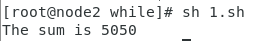3.计算从m加到n的值 #!/bin/bash #计算从m加到n的值 read m read n sum=0 while ((m<=n)) do ((sum+=m)) ((m++)) done echo "The sum is$sum"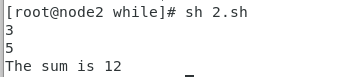4.实现简单加法计算器
实现一个简单加法计算器，用户每行输入一个数字，计算所有数字的和
#!/bin/bash

sum=0
echo "请输入要计算的数字。按下Ctrl+D组合结束读取"

while read n
do
((sum+=n))
done

echo "The sum is $sum "  在终端中读取数据可以等价与在文件中读取数据，按下Ctrl+D组合键表示读取到文件流的末尾，此时read就会读取失败，得到一个非0的退出状态，从而导致判断条件不成立，结束循环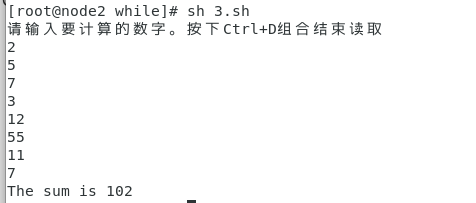展开全文• m为产生不大于n的一个随机数，当产生的随机数mn相等时，退出循环。否则把n加1，再次循环。 运行结果如下： if end条件控制 if后面要一个布尔表达式，后面再跟一个或者多个语句分割，就是一个if..end语句。 ... for循环 循环特定的次数，用end表示循环快结束。示例如下：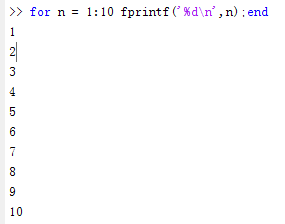注意n可以从1取到10，总共循环10次。 while循环 只要条件为真，就一直循环，用end表示循环快结束。示例如下：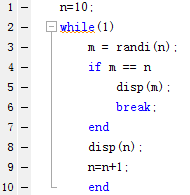该程序中，设置n初值为10，然后进入while循环中。m为产生不大于n的一个随机数，当产生的随机数m和n相等时，退出循环。否则把n加1，再次循环。 运行结果如下：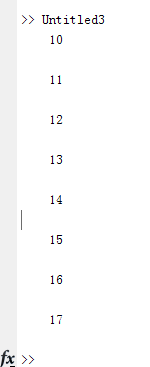if end条件控制 if后面要和一个布尔表达式，后面再跟一个或者多个语句分割，就是一个if..end语句。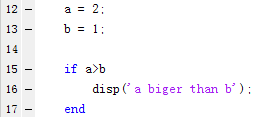运行如下：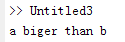if else end 控制语句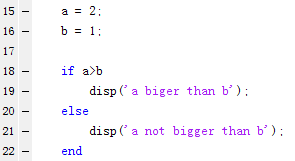运行结果如下：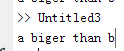if...elseif...elseif...else...end语句 if...elseif...elseif...else...end语句 if 语句可以跟随一个（或多个）可选的elseif..if语句。  展开全文• 1.while循环语句也称为前测试循环语句，利用一个条件来控制是否要继续重复执行这个语句。 语法格式：while(表达式){语句}（必须有循环为假的...示例：计算1一直加到100的和。 var i = 1; var sum = 0; while (i&l 1.while循环语句也称为前测试循环语句，利用一个条件来控制是否要继续重复执行这个语句。 语法格式：while(表达式){语句}（必须有循环为假的情况）。  var n = 1; while (n <= 10){ document.write(n + '\n'); //显示：1 2 3 4 5 6 7 8 9 10 n++; }  示例：计算1一直加到100的和。  var i = 1; var sum = 0; while (i<=100){ sum += i; i++; } document.write("和是" + sum); //显示：和是5050  2.do…while语句：后测试循环语句，利用一个条件来控制是否要继续重复执行这个语句。 语法格式：do{语句}while(表达式);分号要写，不会出错，但是万一有问题呢。  var n = 1; do{ document.write(n + '\n'); //显示：1 2 3 4 5 6 7 8 9 10 n++; }while (n <= 10);  比较：i–的时候比较有问题。 var i = 0; var sum = 0; do{ sum+=i; i++; document.write(i+'\n'); }while (i<=10); document.write('sum='+sum+'||'); var i1 = 0; var sum1 = 0; while(i1<=10){ sum1+=i1; i1++; document.write(i1+'\n'); } document.write('sum1='+sum1); //结果：1 2 3 4 5 6 7 8 9 10 11 sum=55||1 2 3 4 5 6 7 8 9 10 11 sum1=55   展开全文• while 语法： while(循环条件) { 循环体 } 当循环条件为true时，就执行循环代码，否则终止循环； 例如: 用while循环完成计算从1加到n的和 public int sum(int n) { int result =...java • #!/bin/bash # i=0 n=1 //定义循环变量 while [$n -lt 101 ];do //定义循环条件 n < 101 i=$(($i + $n )) ... • 题目描述：求1+2+3+...+n1+2+3+...+n1+2+3+...+n...问题分析：1+2+...+n1+2+...+n1+2+...+n最直观求解方法是(1+n)∗n/2(1+n)*n/2(1+n)∗n/2，考虑题目不允许用乘除法和循环，如何实现“循环加过程呢？可以借... • 1、语法 while语法：当满足某个条件时，就进入循环 ...我们计算1到n加起来的和，用while写脚本016_while.sh： #!/bin/bash if [$# -ne 1 ]; then echo "please input a parameter to caculate" echo "For examplshell
• //没循环一个数就加到sum储存空间里 a++;//自增 } while (sum <= 10000);//循环的条件 Console.WriteLine("最大整数为" + (sum - a) + "最大项为" + (a - 1));//输出 写这个(sum-a)原因是先循环再判断所以他...c#
• //如果j自增平方根还不能除尽，则j加1，说明是质数，此时j=sqrt(i)+1，自动跳出本循环 break; } if (j > sqrt(i) && i != 1) //j=sqrt(i)+1时说明i为质数，符合这个if条件，执行输出语句 ...c++
• 1.判断条件 boolean类型表达式...123# 这个循环是:打印 所有小于等于10数 我们开始先定义了一个变量(n n1) 输出为1 2 3 4 5 6 7 8 9 10 10停止循环# 求0–100 偶数sum=0 n=1 #先来变量 while n&lt...
• 也就是说，我们先得拿： 数字1-数字n 阶乘，然后再把：n个数字阶乘起来，即得到结果； 脑海里搜索回忆以及一番思考后，有三种方法可以解决这道题，以下都写成了方法（也就是函数）形式。 方法一：while循环...java
• An （可能有负数），求A1~An的一个子序列Ai~Aj，使得AiAj的和最大。 解决思想： 要想让时间复杂度为O(N)，就只能用一个for（或while等的）循环。即遍历一遍数组。 1.我们可以从头遍历，把每一个元素相加，每一...子序列 C++ 算法
• 题意：求1加到n的和，要求不能用乘除，以及分支跳转循环如if else while for switch case等语句 思路：这里用到一个巧妙的特性：&& 判断前面不符合就不会执行后面的语句，这样可以在递归的时候不用if就可以...java 算法
• 在第一个GroupBox 中放入3 个TextBox 控件一个Button 按钮，分别用于输入数字、输入加密数字、显示 密后数字计算加密信息；在第二个GroupBox 中放入一个TextBox 控件一个Button 按钮，分别用于显示 解密后...
• #include int main() { void gaid(int number1,int number2); int n,m;...i++) //循环完毕后，把数组中所有值之和加起来，其就是原 游戏者序号 { sum+=arr[i]; } printf("%d",sum); }c 指针
• （一）改错题 序列求和：输入一个正实数eps...错误原因：用do while循环语句在while后面要分号，这个语句特殊需牢记 改正方法：在while后面加上分号 错误信息（2）： 错误原因：item = 1/ n;次语句中n定义为整型...
• 1. while循环 2. for循环 循环n两种写法 for(int i = 0; i < n; ++i){} for(int i = 1; i <= n; ++i){} 3. 循环求和 设置加变量s，记住要将其初始化为0。int s = 0; 循环读入数据，将读入数据加到...c++
• 三行数字中，逗号前面数字是应该要输出，第一次运行结果可以看到是1 2 3 4，这非常明显是上面我手动增加的1，也就意味着在读取第一个换行以后，getline读到的内容长度都是0（但是它又能进循环），第二次跳过直接...c++ ios
• //功能：多项式的加减法操作 #include <iostream.h>//读入必须包含头文件 #include <windows.h>//清屏颜色设置需要 #include #include #include #include <math.h>//处理方幂运算 enum returninfo{...c++
• 题目一 计算1到n的累加 题目描述：求1+2+3+…+n，要求不能使用乘除法、for、...用递归来代替循环，然后从n+n-1开始，一直加到n=0结束。那么问题是，如何判断n！=0呢? 这里便用到一个基本的运算符 “&&...
• 1.在visual studio 2019 中注释错误会直接被系统修改。 2.endl具有换行刷新输出流两个作用。 endl会不停地刷新输出流，频繁操作会降低程序运行效率，这也是C++...//用while循环将50加到100（整数相加） #incluc++
• 顺序表：申请一个MAXSIZE=100大小结构体数组，获取学生个数n，用一个for或者while循环逐个输入学生各项基本信息，并存储结构体中，且每次要记录插入学生人数与上一次操作人数进行加和。 链表：获取学生...
• **第一个奇怪是**，第二个循环 while ((ch_1=getchar())!=toupper(ch_1));//获得名字 本意是想跳过所有小写字母，直到遇到大写字母停止。但是经过运行，只要在名字姓中间空格，无论首字母是否小写，程序都能...c 编程题
• 1. while循环 2. for循环 循环n两种写法 for(int i = 0; i < n; ++i){} for(int i = 1; i <= n; ++i){} 3. 循环求和 设置加和变量s，记住要将其初始化为0 double s = 0; 循环中读入数据 cin>>a; 将...c++
• 1. while循环 2. for循环 循环n两种写法 for(int i = 0; i < n; ++i){} for(int i = 1; i <= n; ++i){} 3. 循环求和 设置加变量s，记住要将其初始化为0。int s = 0; 循环读入数据，将读入数据加到...c++...